# 1 Introduction

The following tutorial outlines the use of the ELEFAN functions available in TropFishR (Tobias K. Mildenberger, Taylor, and Wolff 2017; Tobias Karl Mildenberger, Taylor, and Wolff 2017) (ELEFAN, ELEFAN_GA, and ELEFAN_SA). These functions can be used to estimate growth model parameters from length-frequency (lfq) data. The ability to convert length to relative age is the initial step in length-based stock assessment, and underpins subsequent analyses (see the TropFishR tutorial for a broader overview of length-based stock assessment).

The growth model presently implemented within TropFishR is the von Bertalanffy growth function (VBGF),

$L_{t} = L_{\infty} (1-\exp(-K(t-t_0))),$

where $$L_t$$ is length-at-age $$t$$, $$L_\infty$$ is asymptotic length, $$K$$ is the von Bertalanffy growth constant, and $$t_0$$ is the theoretical age when length equals zero. $$t_0$$ is usually negative, resulting in a positive length at the time of recruitment (t = 0). In TropFishR, these parameters are referred to as Linf, K, and t0.

The following plot shows the basic form of the VBGF under varying K settings:

library(TropFishR)
t <- seq(-0.2, 3, length.out = 200)
K <- c(2, 1, 0.5)
COL <- rep(1,3)
LTY <- 1:3
for(i in seq(K)){
Lt <- VBGF(param = list(Linf = 20, K = K[i], t0 = -0.1), t = t)
if(i == 1){
plot(t, Lt, t="l", ylim = c(0,22), yaxs="i", col = COL[i], lty = LTY[i])
abline(v = 0, col = 8, lty = 3)
abline(h = 20, col = 3, lty = 3)
points(x = -0.1, y = 0, pch = 16, col = 4)
text(x = -0.1, y = 0, labels = expression(italic(t)), adj=c(1,-0.5), col=4)
text(x = -0.1, y = 20, labels = expression(italic(L[infinity])), adj=c(1,-0.5), col=3)
legend("bottomright", legend = paste("K =", K), lty=LTY, col=COL, bty="n")
}else{
lines(t, Lt, col = COL[i], lty = LTY[i])
}
}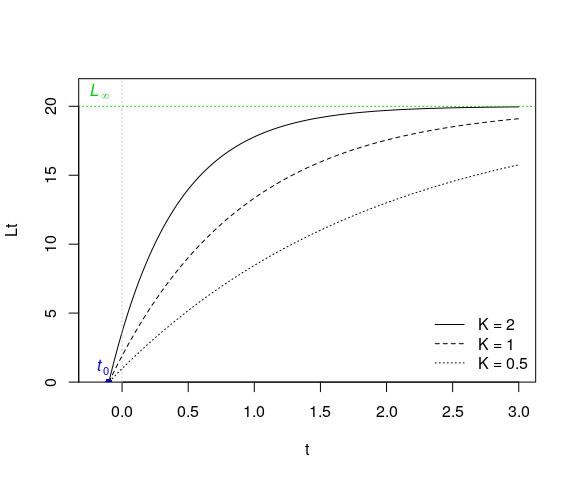TropFishR also offers users the ability to model inter-annual variability in growth via a seasonally-oscillating VBGF (soVBGF),

$L_{t} = L_{\infty} (1-\exp(-(K(t-t_0)+S(t)-S(t_0)))),$

where $$S(t) = (CK/2\pi)\sin 2\pi(t−t_s)$$, $$C$$ is a constant indicating the amplitude of the oscillation, typically ranging from 0 to 1 (a value $$>1$$ implies periods of shrinkage, which is rare), and $$t_s$$ is the fraction of a year (relative to the age of recruitment, $$t=0$$) where the sine wave oscillation begins (i.e. turns positive). In TropFishR, these additional oscillation parameters are written as C and ts.

The following plot demonstrates the effect of variable $$C$$ settings:

library(TropFishR)
t <- seq(-0.2, 3, length.out = 200)
Lt <- VBGF(param = list(Linf = 20, K = 1, t0 = -0.1, ts = 0, C=0), t = t)
Cs <- seq(0.25,1,0.25)
COLs <- 1:5
plot(t, Lt, t="l", ylim=c(0,22), yaxs="i", col=1)
for(i in seq(Cs)){
lines(t, VBGF(param = list(Linf = 20, K = 1, t0 = -0.1, ts = 0, C=Cs[i]), t = t), col=COLs[i+1])
}
legend("bottomright", legend=paste("C =", c(0,Cs)), lty=1, col=COLs, bty="n")
abline(v=0, col = 8, lty = 3)
abline(h = 20, col = 8, lty=3)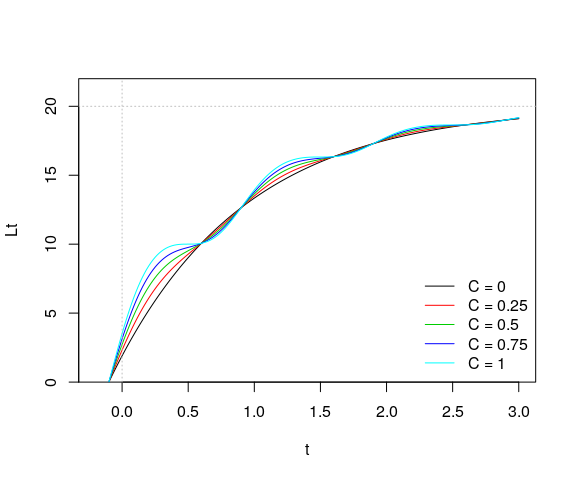Such variation in growth is common in many organisms, but may be more pronounced in temperate areas where intra-annual environmental variability is of larger magnitude (e.g. temperature, primary production).

# 2 Length-frequency data preparation

The first step to using the ELEFAN functions is to have a length-frequency object loaded (class lfq). For the majority of the following examples, we will use the relatively small alba lfq dataset (Abra alba mollusc sampled in Kiel Bay (Brey, Soriano, and Pauly 1988)), which consists of approximately bi-monthly sampling of length-frequencies taken over the course of one and a half years. The alba dataset is included within the current TropFishR version.

An lfq class dataset is essentially a list containing the following elements: a catch frequency matrix (lfq$catch), a vector of mid-lengths (lfq$midLengths) corresponding to rows of the catch matrix, and a vector of dates (lfq$dates) corresponding to the columns of the catch matrix. The lfq class is used by several functions within the TropFishR package (e.g. plot.lfq). A separate tutorial exists detailing the creation of such objects from scratch (link), but here is a small example that passes variables from alba to a new lfq object. First, the three elements are added to a list: data("alba") tmplfq <- list( midLengths = alba$midLengths,
dates = alba$dates, catch = alba$catch
)

Finally, we need to assign the class lfq to our new object in order to allow it to be recognized by other TropFishR functions, e.g. plot.lfq:

class(tmplfq) <- "lfq"
plot(tmplfq, Fname="catch", hist.sc = 1)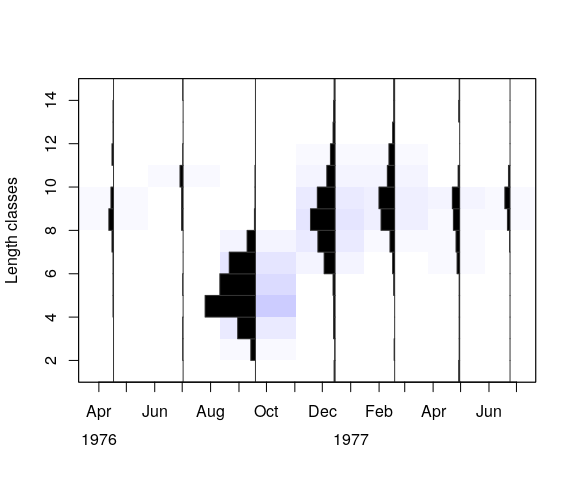# 3 The ELEFAN approach

## 3.1 Data restructuring

Before proceeding with the ELEFAN functions, one should first explore settings for the restructuring of lfq values. Restructuring calculates a score for each lfq bin by comparing the count value against the moving average (MA) across neighboring bins. The default setting of MA = 5, as is used in FiSAT II, compares each bin with the average across 5 bins (i.e. +/- 2 bins to either side). As was shown by Taylor and Mildenberger (2017), the MA setting used can significantly affect the scoring of growth curves during the fitting process. As a rule-of-thumb, it is suggested that the MA setting should approximate the number of bins spanning the smallest (i.e. youngest) cohort width. In the alba dataset, the smallest cohort is that which appears in the lfq data in mid-September, and its numbers are predominantly in 6 bins (from sizes 3-7 cm), plus 2 bins on each side with lower counts. Since the MA setting must be an odd-number, MA = 7 seems to be an appropriate setting. It should be noted that while FiSAT II does not allow for changing the MA setting, one still has the option to adjust the bin width, which could be used to aggregate counts into a smaller number of bins more in line with the MA = 5 moving average setting. The following plot shows reconstructed frequencies, with negative and positive values as white and black colored histograms, respectively. Background coloring is added to help visualize sign and magnitude of the bin values.

alba <- lfqRestructure(alba, MA = 7)
plot(alba, hist.sc = 0.75)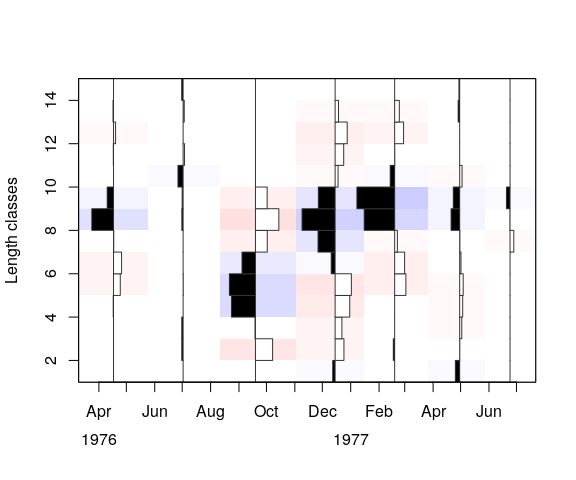## 3.2 Scoring of VGBF parameter combinations

Depending on the restructuring settings, the distribution and amplitude of positive and negative scoring bins will be affected. The scoring procedure used in TropFishR follows that of FiSAT II, as outlined by Gayanilo and Pauly (1997). For a given set of VBGF parameters, yearly repeating growth curves are drawn through the lfq data. Scoring is based on the concept of “peaks”, which are runs of positive or negative scoring bins. Each peak takes the value of the largest absolute value contained within.

The sum of all positive peaks is called the “available sum of peaks” (ASP), which represents a maximum possible score (if no negative bins are crossed). The “estimated sum of peaks” (ESP) is the sum of peak values crossed by the growth curves, with the caveat that positively crossed bins are only counted once (i.e. “flagged out”) while negatively crossed bins are counted every time they are hit (Pauly 1985).

Finally, as a measure of relative fit, the ratio of the two values is used to calculate “new R” (Rn), which can have a maximum value of 1.0:

$Rn = 10^{(ESP/ASP)}/10$

In the following plot, background shading shows runs of peaks, with positive peaks in blue, negative peaks in red, and values of zero in white.

alba <- lfqRestructure(alba, MA=7)
plot(alba, hist.col = c("white", "black"),
image.col = c(rep(rgb(1,0.8,0.8),1000), "white", rep(rgb(0.8,0.8,1),1000)),
ylim = c(0,max(alba$midLengths+0.5))) tmp <- lfqFitCurves(alba, par = list(Linf=11, K=2.5, t_anchor=0.5), draw = TRUE, col=4, lty=2)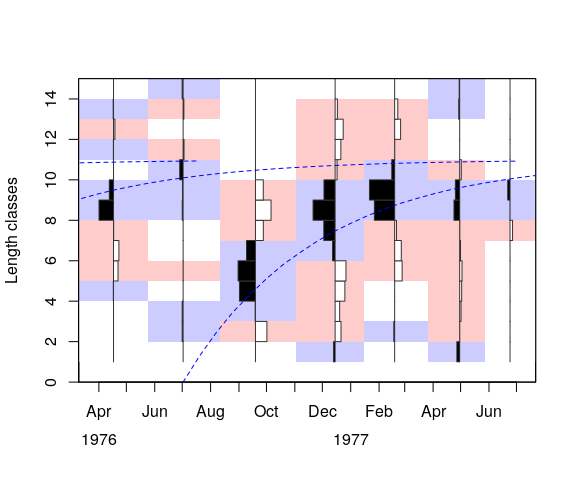In ELEFAN, time is used rather than age, and the point where the growth curve crosses length zero is referred to as the “anchor time” (t_anchor). This is then interpreted as the time when length equals zero. In the above plot,t_anchor = 0.5, which corresponds to halfway through the year (i.e. July 1st). Likewise, the meaning of ts is slightly different; it is the time of the year when growth turns positive. In some ways, the shift towards a time perspective can help provide important biological information (i.e. identify season when growth strongest). Furthermore, back-calculation of length zero across bins allows for the reconstruction of a relative recruitment index (see ?recruitment). # 4 Restricting the VBGF parameter search range ## 4.1 Asymptotic length (Linf) Linf has been a historically difficult parameter to estimate with ELEFAN due to low counts at large sizes. One recommendation is to approximate Linf outside of ELEFAN, e.g. via a Powell-Wetherall plot, which may help to refine searches to a smaller range of possible values. We will see later that several maxima (high scoring regions) of the parameter search space can make the selection of a “best” combination difficult, so searches restricted to sensible values is recommended. TropFishR includes the function powell_wetherall, which can be used to estimate Linf, including confidence intervals. The selection of length classes to include in the regression should be restricted to fully-exploited sizes that contain relatively large frequencies. In this example, both the smallest and largest sizes have been excluded, which results in Linf = 11.74cm and a confidence interval between 9.90 and 13.58cm. PW <- powell_wetherall(alba, catch_columns = 1:7, reg_int = c(2,9) )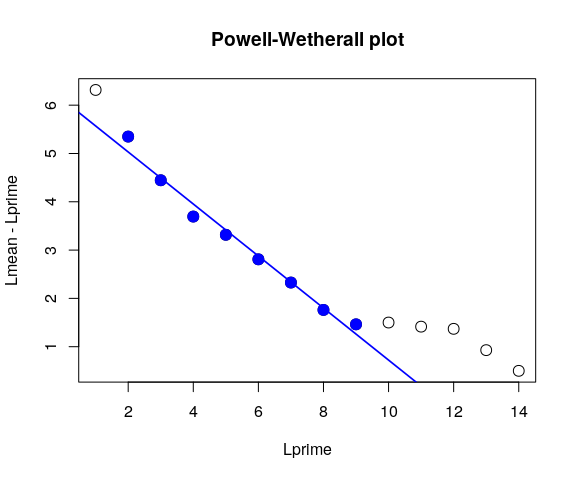PW$Linf_est
##  11.3413
PW$confidenceInt_Linf ##  8.977609 13.704995 However, the Powell-Wetherall approach has been criticized for providing unreliable results in cases where lfq data spans a small range of length classes, or in severely depleted populations where large individuals are rare. Therefore, the results should be used with caution. Another approach may be to inform first guesstimates of Linf from other data sources, and allow ELEFAN a significant range of values for searching during VBGF fitting. Froese and Binohlan (2000) also showed strong correlation between the largest individuals (Lmax) and Linf, $\log(L_\infty) = 0.044 + 0.9841 * \log(L_{max})$ , but Lmax may also be difficult to obtain in highly exploited populations. ## 4.2 Growth constant (K) Following limiting the range for Linf, K is likely to be restricted automatically based on the quality of the lfq data. Nevertheless, viewing possible K values for similar species, or for the same species in other studies, may allow for restrictions in the search range. Generally, small, short-lived species will have higher values in K (e.g. > 1.5), while large, longer-lived species will have lower values (< 1.0). ## 4.3 Response surface analysis (RSA) As with FiSAT II, TropFishR allows one to visualize fitting scores across a range of Linf and K combinations in a so-called Response surface analysis (RSA). This provides a nice visualization of Rn scores across discrete combinations of both variables, and may also aid in narrowing possible variable ranges in subsequent, more refined searches. In TropFishR, RSA is conducted using the ELEFAN function. Specifically, a sequence of Linf and K values are provided to the arguments Linf_range and K_range. All combinations of those parameters are used, and the best scoring t_anchor is solved for. If a prominent cohort can be identified, one can force the VBGF to cross through a defined bin, using the argument setting method = "cross", which will substantially reduce the computation time. alba2 <- ELEFAN( lfq = alba, MA = 7, Linf_range = seq(7, 20, length.out = 30), K_range = exp(seq(log(0.1),log(4), length.out = 30)), method = "cross", cross.date = alba$dates,
cross.midLength = alba$midLengths, contour = TRUE, add.values = FALSE, hide.progressbar = TRUE # change to 'TRUE' to follow algorithm's progression ) ## Optimisation procuedure of ELEFAN is running. ## This will take some time. ## The process bar will inform you about the process of the calculations. points(alba2$par["Linf"], alba2$par["K"], pch="*", cex=2, col=2)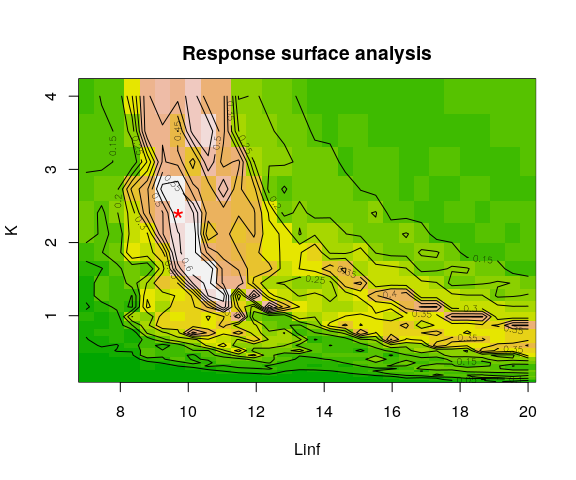unlist(alba2$par)
##     Linf        K t_anchor        C       ts     phiL
## 9.689655 2.400000 0.370000 0.000000 0.000000 2.352828
alba2$Rn_max ##  0.649 In the above plot, the highest Rn score is denoted by a red star symbol(*), although there exists a larger area of relatively similar scores. This is typical of an RSA plot, where a plateau of higher scores often forms along a banana-shaped area of negatively correlated K and Linf values, which generally follows an isocline of the growth performance index ($$\phi' = \log(K)+ 2\log(L_{\infty})$$). In the best case, a single maxima will stand out, revealing the best parameter combination, however this is usually rare. Nevertheless, a wide range of values can be visualized, allowing for reduced ranges in subsequent RSAs or refined searches via other algorithms (see following section below). The resulting best scoring combination is added to the lfq object in the par element, allowing for direct visualization via plot.lfq. In the following plot, the bin designated for crossing is denoted by a red star symbol(*): plot(alba2) points(alba$dates, alba$midLengths, pch="*", cex=2, col=2)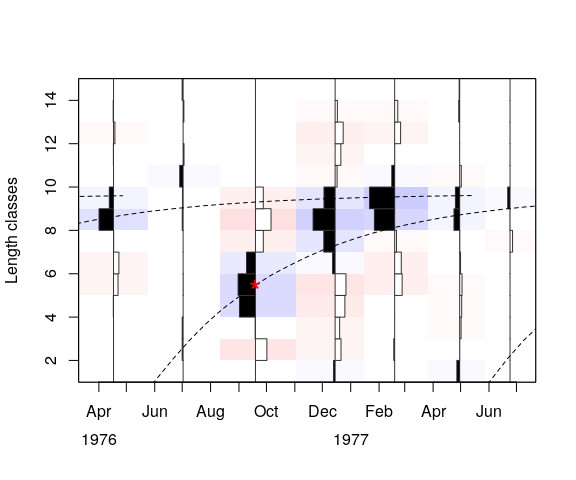# 5 Refined searches using optimization algorithms TropFishR contains two functions that allow for more refined searches of VBGF parameters: ELEFAN_SA and ELEFAN_GA. In both cases, VBGF parameters are fit on a continuous scale, allowing for more precise estimates. Both methods require that the user specify limits to the VBGF parameters in order to constrain the search space. The confidence intervals obtained from the P-W plot, as well as a visual inspection of higher Rn values in the RSA, helped define these ranges in the examples below. ## 5.1 Simulated annealing (ELEFAN_SA) The first optimization algorithm offered in TropFishR is called “simulated annealing” (ELEFAN_SA). The simulated annealing algorithm begins with a highly randomized search, which gradually focuses on regions of the parameter search space with highest Rn scores. The degree of the initial randomized searching will depend on the starting temperature (SA_temp), while the stopping time can be controlled by either the maximum calculation time (SA_time) or maximum number of iterations (maxit). For reproducibility across computing platforms, maxit is the more appropriate stopping point. set.seed(1) alba3 <- ELEFAN_SA( lfq = alba, seasonalised = FALSE, init_par = alba2$par[1:5],
low_par = list(Linf=PW$confidenceInt_Linf, K=1, t_anchor=0, ts=0, C=0), up_par = list(Linf=PW$confidenceInt_Linf, K=4, t_anchor=1, ts=1, C=1),
SA_temp = 2e5,
SA_time = 60,
maxit = 400,
MA = 7,
plot.score = TRUE,
verbose = FALSE
)
## Simulated annealing is running.
## This will take approximately 1 minutes.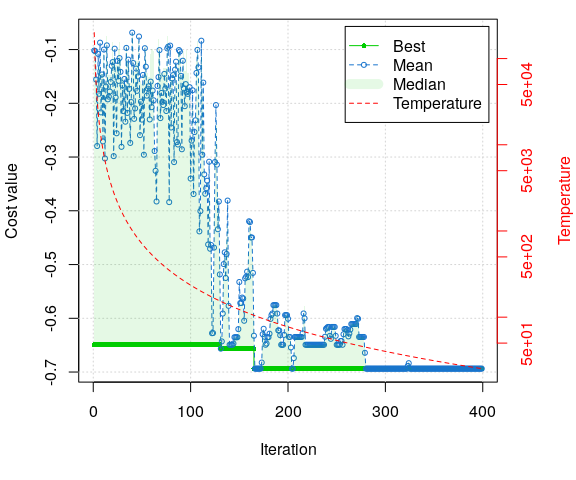unlist(alba3$par) ## Linf K t_anchor phiL ## 10.1948485 1.8905354 0.2499204 2.2933464 alba3$Rn_max
##  0.6937336

It is advised to use an SA_temp setting that allows for a period of random searching, before more refined local searching begins. In the above example, the more random search behavior is observed during the initial 100 iterations, and no improvements to Rn were found after ca. 170 iterations.

Again, the resulting best scoring parameter combination is added to $par, and can be directly plotted: plot(alba3)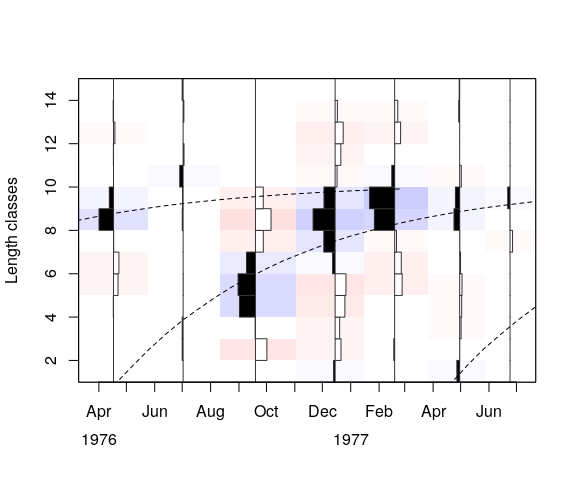## 5.2 Genetic algorithm (ELEFAN_GA) The second optimization algorithm offered in TropFishR is called “genetic algorithm” (ELEFAN_GA). The genetic algorithm uses the idea of natural selection to find best scoring parameter combinations. Parameters are treated like genes carried by individuals in the population carry. Individuals that have the highest fitness (i.e. Rn score) reproduce and recombine their parameter values in subsequent generations. Over time, the population will come to be dominated by individuals that have ever increasing fitness. As with ELEFAN_SA, the initial generations have parameters chosen at random within the defined intervals. Over time, the variability in the parameters gradually decreases becomes more focused on local maxima. Important settings include the number of individuals in the population (popSize), the probability of mutation in the parent gene (pmutation), the maximum number generations (maxiter), and, as a stopping rule, the maximum number of generations without improvement (run). The pmutation setting is usually set low, although higher values will increase the randomness of the search. Increasing popSize will increase the number of starting parameter combinations in the population, which will both increase the initial coverage of the parameter space as well as effect the degree of localized searching during later generations. set.seed(1) alba4 <- ELEFAN_GA( lfq = alba, seasonalised = FALSE, low_par = list(Linf=PW$confidenceInt_Linf, K=1, t_anchor=0, ts=0, C=0),
up_par = list(Linf=PW$confidenceInt_Linf, K=4, t_anchor=1, ts=1, C=1), popSize = 60, pmutation = 0.2, maxiter = 100, run = 20, MA = 7, plot.score = TRUE, monitor = FALSE, parallel = FALSE ) ## Genetic algorithm is running. This might take some time.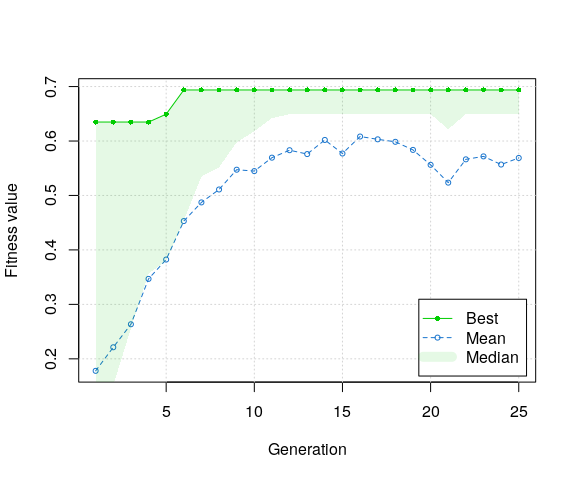unlist(alba4$par)
##       Linf          K   t_anchor       phiL
## 10.1301838  1.9618729  0.2643963  2.3039055
alba4$Rn_max ##  0.6937336 As with ELEFAN_SA it is important to review the plot of Rn scores by generation in order to check the algorithm’s performance. In this example, a best solution is achieved after only 5 generations with 60 individuals. Extending the run argument will also affect the degree to which local maxima are searched during later generations. One advantage of ELEFAN_GA over ELEFAN_SA is that the search can be done with parallelized computation (argument parallel = TRUE), which can result in faster solutions. Again, the best fitting combination is added to $par and the results can be directly plotted over the lfq data:

plot(alba4)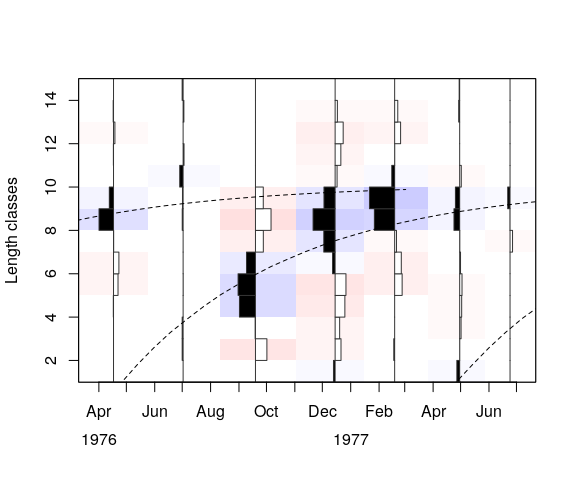## 5.3 Seasonally-oscillating growth

The traditional ELEFAN functions also offer searching based on the soVBGF, although these values must be fixed (e.g. in RSA), and thus their estimation must be derived from other methods. Including all 5 possible parameter combinations of the soVBGF (Linf, K, t_anchor, C, and ts) would require an exponentially higher number of calculations, making the approach impractical. To the contrary, both ELEFAN_SA and ELEFAN_GA allow for easy incorporation of these parameters in a more optimized search. Again, algorithms search for all parameters along a continuous scale.

The following examples demonstrate fitting of soVBGF to both alba and a synthetically-generated lfq dataset (synLFQ4) using ELEFAN_GA. The main additions to the functions arguments are to set seasonalized = TRUE and to include lower and upper bounds for ts and C in low_par and up_par, respectively. Unless there is evidence to restrict these parameters, bounds spanning the full range of the parameters (i.e. between 0 and 1) are recommended.

### 5.3.1 alba

set.seed(1)
alba5 <- ELEFAN_GA(
lfq = alba,
seasonalised = TRUE,
low_par = list(Linf=PW$confidenceInt_Linf, K=0.1, t_anchor=0, ts=0, C=0), up_par = list(Linf=PW$confidenceInt_Linf, K=4, t_anchor=1, ts=1, C=1),
popSize = 60,
pmutation = 0.2,
maxiter = 100,
run = 20,
MA = 7,
plot.score = TRUE,
monitor = FALSE,
parallel = FALSE
)
## Genetic algorithm is running. This might take some time.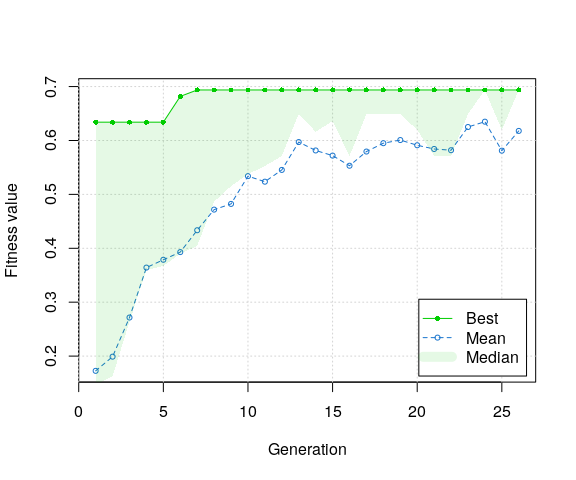unlist(alba5$par) ## Linf K t_anchor C ts phiL ## 10.2363568 1.8799341 0.2350472 0.3332832 0.7712340 2.2944335 alba5$Rn_max
##  0.6937336
plot(alba5)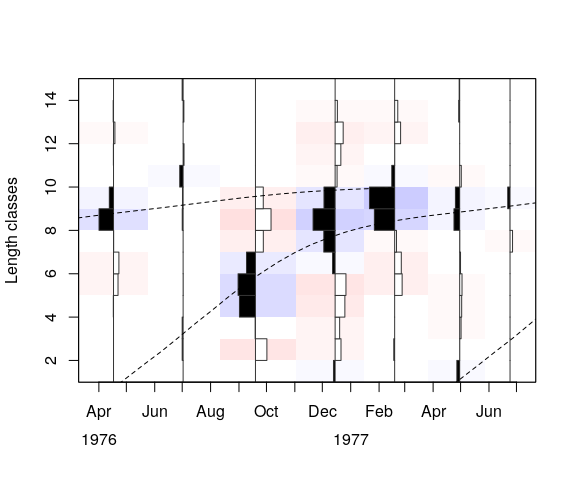In the case of the alba dataset, no real improvement in Rn is observed for the soVBGF fit (alba5$Rn_max) over that of the VBGF fit (alba4$Rn_max). Although no statistical test is (yet) available for selecting one model over the other, the lack of sufficient improvement would seem to suggest selecting the simpler VBGF model.

### 5.3.2 A synthetic example

For the synthetic lfq dataset synLFQ4, the soVBGF parameters used in its generation are known:

true_par <- list(Linf = 80, K = 0.5, t_anchor = 0.25,C = 0.75,  ts = 0.5, phiL = 3.51)

The dataset is significantly more detailed (72 length bins, 25 time samples) than alba (14 length bins, 7 time samples), and thus will take longer to solve. For such large lfq data sets, solution time may be reduced through use of parallel computing (i.e. parallel = TRUE). From the previous work presented by Taylor and Mildenberger (2017), we know that a moving average setting of MA = 11 is appropriate for this lfq data.

set.seed(1)
synLFQ4 <- ELEFAN_GA(
lfq = synLFQ4,
seasonalised = TRUE,
low_par = list(Linf=70, K=0.1, t_anchor=0, ts=0, C=0),
up_par = list(Linf=110, K=1, t_anchor=1, ts=1, C=1),
popSize = 60,
pmutation = 0.2,
maxiter = 100,
run = 20,
MA = 11,
plot.score = TRUE,
monitor = FALSE,
parallel = FALSE
)
## Genetic algorithm is running. This might take some time.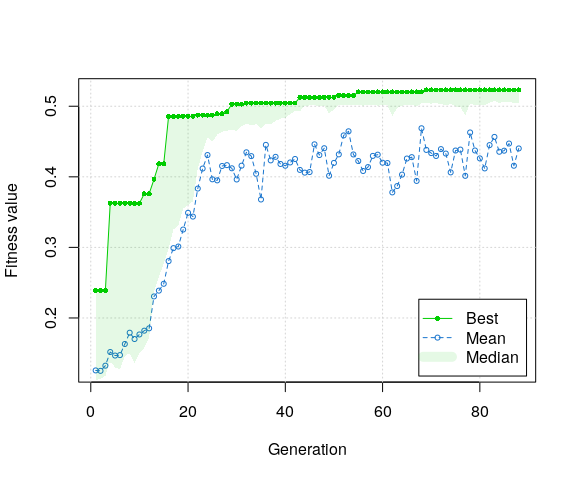In this case, the true values are very well estimated:

tmp <- as.data.frame(rbind(unlist(true_par), unlist(synLFQ4$par))) rownames(tmp) <- c("true", "estimated") tmp$Rn <- c(synLFQ4$Rn_max, lfqFitCurves(synLFQ4, par = true_par)$Rn_max)
tmp <- round(tmp,3)
tmp
Linf K t_anchor C ts phiL Rn
true 80.000 0.50 0.250 0.750 0.500 3.510 0.523
estimated 86.541 0.41 0.086 0.732 0.514 3.487 0.453
plot(synLFQ4, draw = FALSE)
tmp <- lfqFitCurves(synLFQ4, par = true_par, col=8, lty=1, draw = TRUE)
tmp <- lfqFitCurves(synLFQ4, par = synLFQ4\$par, col=4, lty=2, draw = TRUE)
legend("top", ncol=2, legend = c("true", "estimated"), col=c(8,4), lty=c(1,2))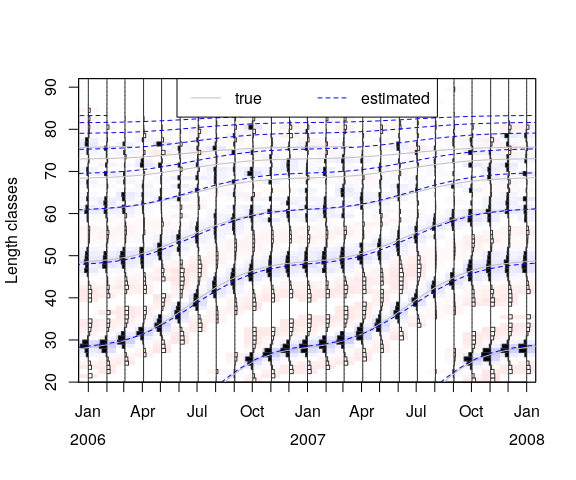## 5.4 Variable mutation probability

In most cases, using constant mutation probability setting (pmutation) is sufficient to achieve a good fit with ELEFAN_GA. Nevertheless, it may be desired to use a variable setting to adjust the periods of random versus focused searching. The GA package has a built in function (ga_pmutation) that allows for a decreasing pmutation rate over the iteration time (see ?GA::pmutation for further information).

The following example uses ELEFAN_GA with a variable mutation rate, which starts at 0.5 and decreases to 0.1. This gradual focusing of pmutation rates in a way mimics the decreasing temperature of simulated annealing.

synLFQ4 <- ELEFAN_GA(
lfq = synLFQ4,
seasonalised = TRUE,
low_par = list(Linf=70, K=0.1, t_anchor=0, ts=0, C=0),
up_par = list(Linf=110, K=1, t_anchor=1, ts=1, C=1),
popSize = 60,
pmutation = function(...) GA::ga_pmutation(..., p0=0.5, p=0.1),
maxiter = 100,
run = 20,
MA = 11,
plot.score = TRUE,
monitor = FALSE,
parallel = FALSE
)
## Genetic algorithm is running. This might take some time.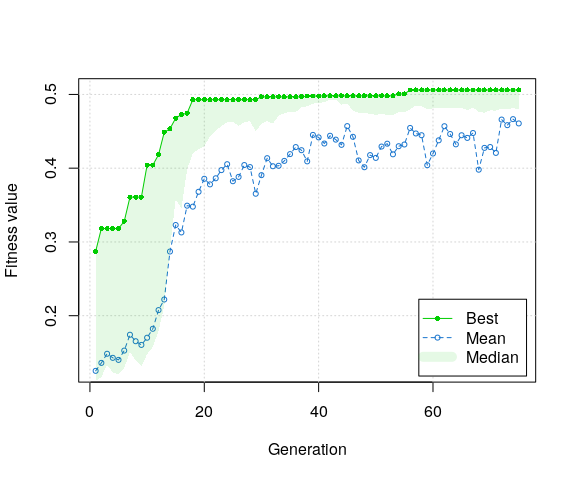# 6 Best practices

The following list highlights some existing best practices for using the ELEFAN approach:

• lfq data
• should contain relatively high counts that are representative of the length distribution of the population (in the best case) or catches.
• should be sampled at regular intervals throughout the year, and at roughly the same sampling effort.
• should cover a substantial degree of small length classes, as their relatively higher numbers and more apparent growth will aid in the fitting of the VBGF. A general rule-of-thumb could be that the smallest bins should start at at least 25% of Linf.
• MAsetting
• should approximate the number of bins spanning the width of a cohort following recruitment.
• Optimization settings
• should allow for sufficient coverage of the full parameter search space during the initial, more randomized part of search (i.e. SA_temp argument inELEFAN_SA; popSize and pmutation arguments in ELEFAN_GA).
• should allow for sufficient search time during later iterations / generations so that parameter space containing local maxima are fully explored (i.e. SA_time and maxit arguments inELEFAN_SA; maxiter, elitism, and run arguments in ELEFAN_GA).